L1. Freely falling mass
1/h
i
i
i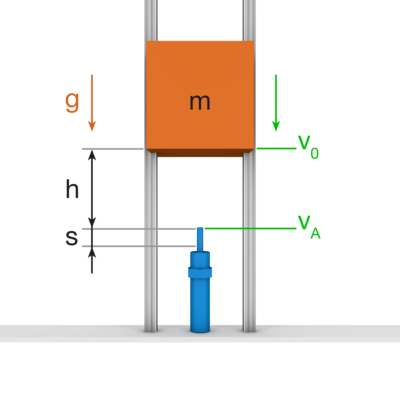L2. Downwards moving mass with counteracting driving force
N
1/h
i
i
i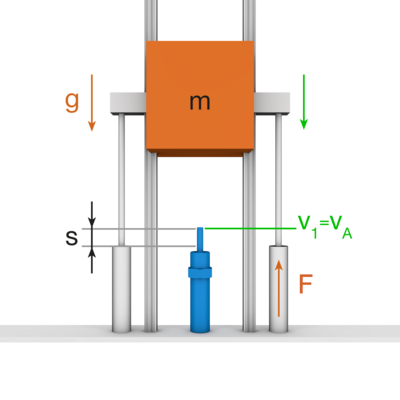L3. Downwards moving mass with driving force
N
1/h
i
i
i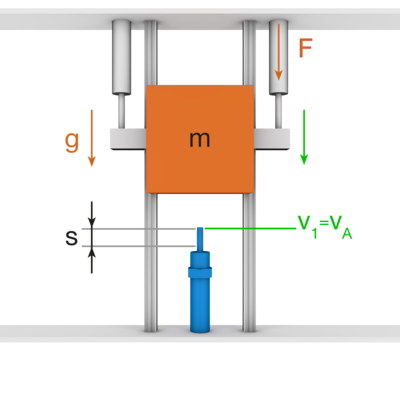L4. Upwards moving mass with driving force
N
1/h
i
i
i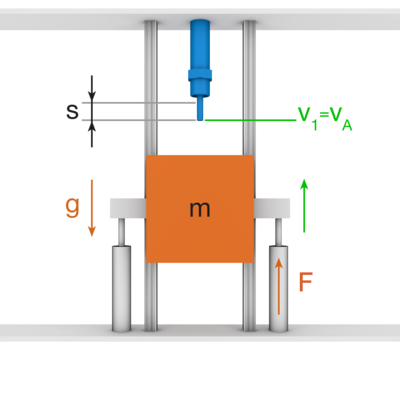L5. Horizontally moving mass without driving force
1/h
i
i
i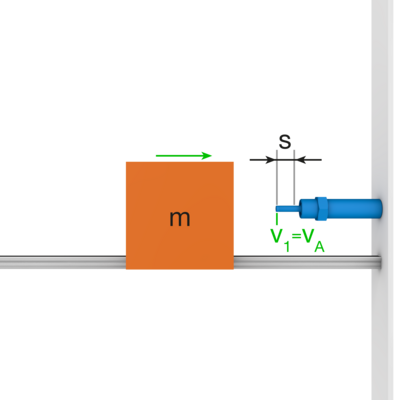L6. Horizontally moving mass with form fit driving force
N
1/h
i
i
i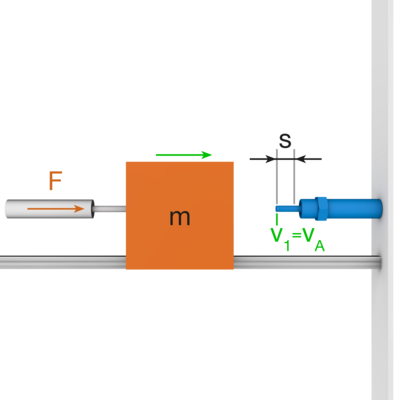L7. Horizontally moving mass with frictional driving force
1/h
i
i
i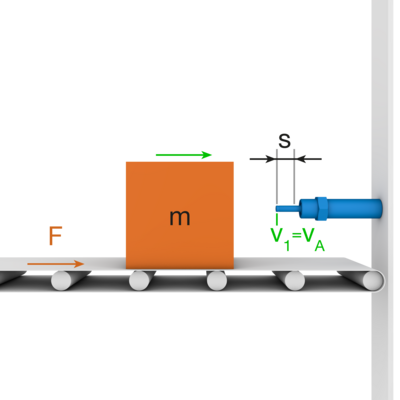L8. Falling mass on an inclined plane
°
1/h
i
i
i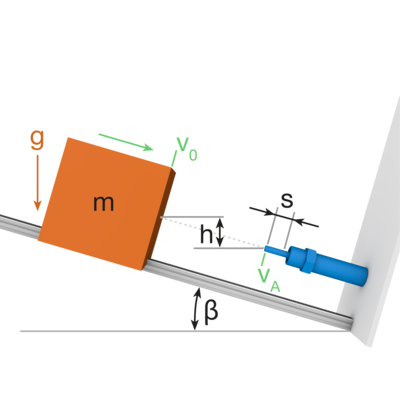L9. Downward moving mass with opposing drive force on an inclined plane
N
°
1/h
i
i
i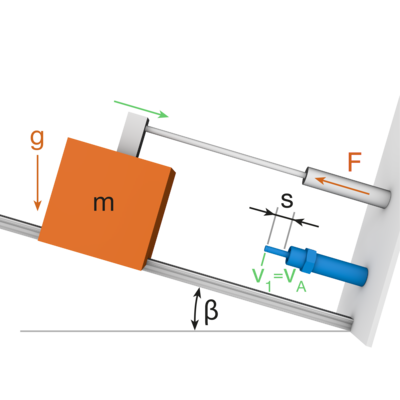L10. Downward moving mass with drive force on an inclined plane
N
°
1/h
i
i
i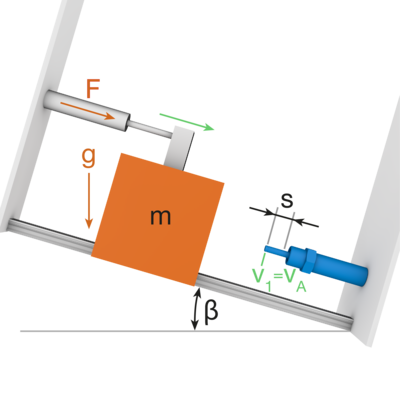L11. Upward moving mass with drive force on an inclined plane
N
°
1/h
i
i
i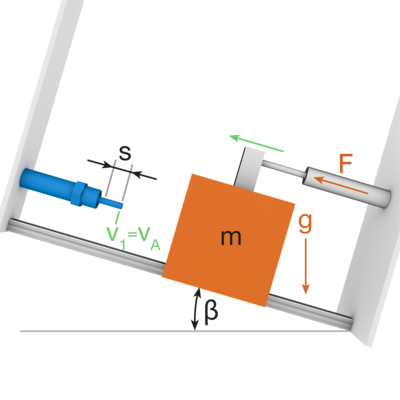R1a. Freely oscillating mass at horizontal impact
Calculation according to rotational parameters
kg*m²
1/s
1/h
i
i
i
Simplified calculation according to translational parameters
1/h
i
i
i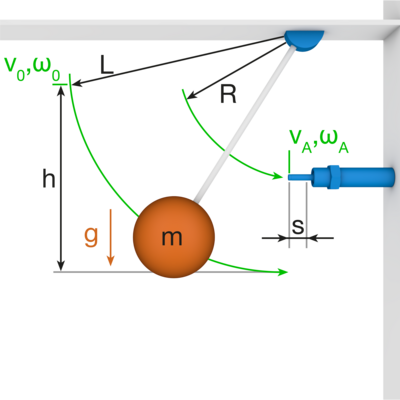R1b. Freely oscillating mass at vertical impact
Calculation according to rotational parameters
kg*m²
1/s
1/h
i
i
i
Simplified calculation according to translational parameters
1/h
i
i
i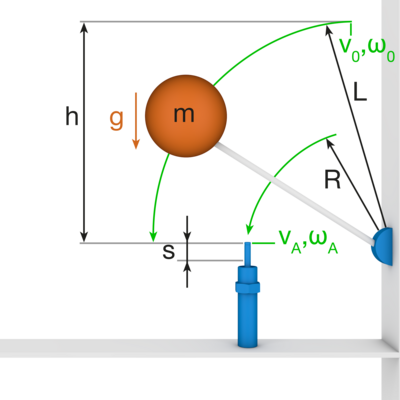R2a. Downwards pivoting mass with counteracting driving torque at horizontal impact
Calculation according to rotational parameters
kg*m²
1/s
1/h
i
i
i
Simplified calculation according to translational parameters
1/h
i
i
i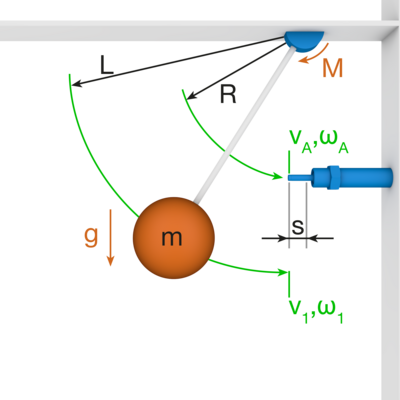R2b. Downwards pivoting mass with counteracting driving torque at vertical impact
Calculation according to rotational parameters
kg*m²
1/s
1/h
i
i
i
Simplified calculation according to translational parameters
1/h
i
i
i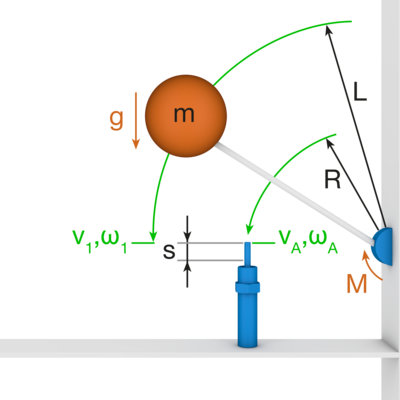R3a. Downwards pivoting mass with driving torque at horizontal impact
Calculation according to rotational parameters
kg*m²
1/s
1/h
i
i
i
Simplified calculation according to translational parameters
1/h
i
i
i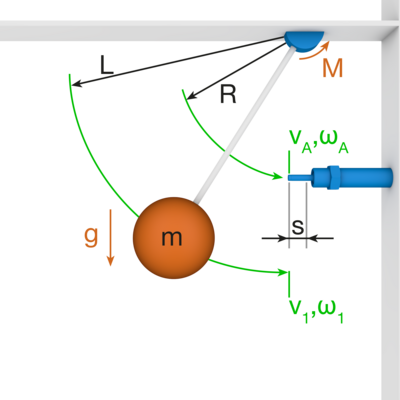R3b. Downwards pivoting mass with driving torque at vertical impact
Calculation according to rotational parameters
kg*m²
1/s
1/h
i
i
i
Simplified calculation according to translational parameters
1/h
i
i
i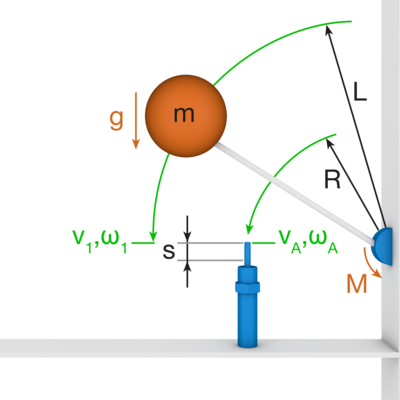R4a. Upwards pivoting mass with driving torque at horizontal impact
Calculation according to rotational parameters
kg*m²
1/s
1/h
i
i
i
Simplified calculation according to translational parameters
1/h
i
i
i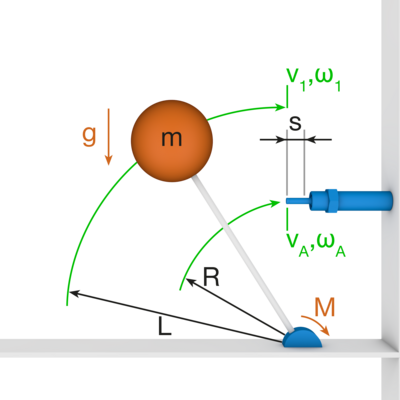R4b. Upwards pivoting mass with driving torque at vertical impact
Calculation according to rotational parameters
kg*m²
1/s
1/h
i
i
i
Simplified calculation according to translational parameters
1/h
i
i
i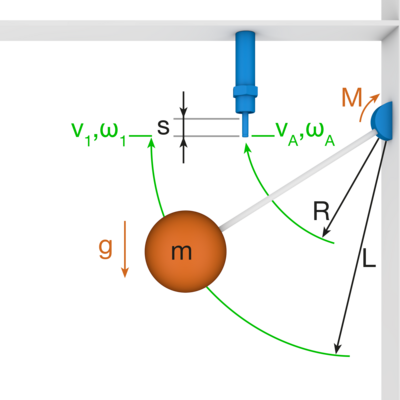R5. Horizontally pivoting mass without driving torque
Calculation according to rotational parameters
kg*m²
1/s
1/h
i
i
i
Simplified calculation according to translational parameters
1/h
i
i
i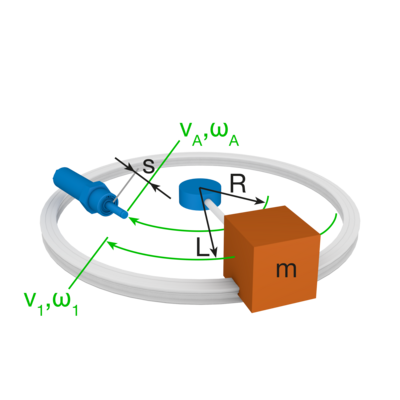R6. Horizontally pivoting mass with form fit driving torque
Calculation according to rotational parameters
kg*m²
1/s
1/h
i
i
i
Simplified calculation according to translational parameters
1/h
i
i
i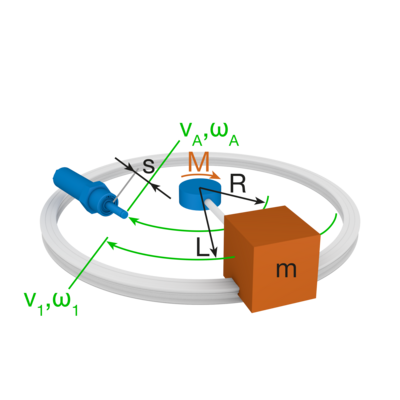R7. Horizontally pivoting mass with frictional driving torque
Calculation according to rotational parameters
kg*m²
1/s
1/h
i
i
i
Simplified calculation according to translational parameters
1/h
i
i
i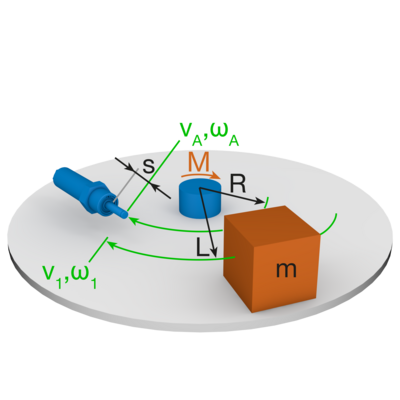Conversion of effective mass to velocity
Shock absorber data
Nm
kg
kg
Velocity range
m/s
m/s

Conversion of velocity to effective mass
Shock absorber data
Nm
m/s
m/s
Range of effective mass
kg
kg
Results
Please enlarge/change your filter selection so that the filter can be applied